## Definition

A graded ring is a commutative unital ring$A$ equipped with a direct sum decomposition as a sum of Abelian subgroups:$A = \oplus_{i=-\infty}^\infty A_i = \cdots A_{-2} \oplus A_{-1} \oplus A_0 \oplus A_1 \oplus A_2 \oplus \cdots$

such that the following hold:

• Each$A_i$ is a subgroup under addition
•$1 \in A_0$
•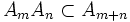$A_mA_n \subset A_{m+n}$. In other words, if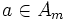$a \in A_m$ and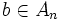$b \in A_n$ then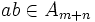$ab \in A_{m+n}$

A structure of the above sort on a ring is termed a gradation, also a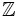$\mathbb{Z}$-gradation. The ring$A$ is positively graded if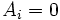$A_i = 0$ for all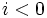$i<0$.

There are related notions for noncommutative rings.